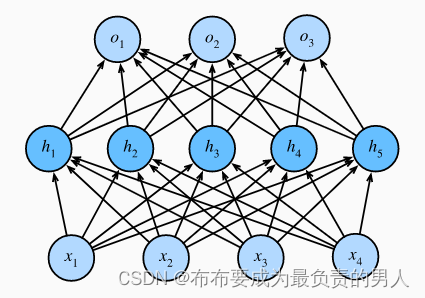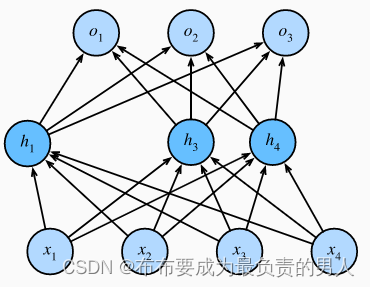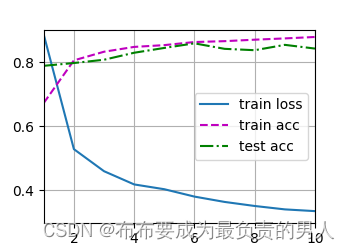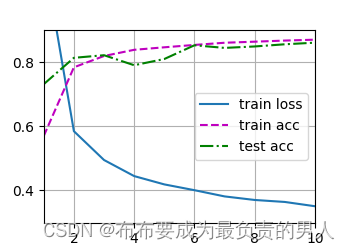## 机器学习深度学习——Dropout

chatgpt/2023/10/4 8:57:08

👨‍🎓作者简介：一位即将上大四，正专攻机器学习的保研er
🌌上期文章：机器学习&&深度学习——权重衰减
📚订阅专栏：机器学习&&深度学习

• 重新审视过拟合
• 扰动的稳健性
• 实践中的Dropout
• 从零开始实现
• 定义模型参数
• 定义模型
• 训练和测试
• 简洁实现
• 小结

# 扰动的稳健性

h ′ = { 0 ，概率为 p h 1 − p ，其他情况 h^{'}= \begin{cases} \begin{aligned} 0，概率为p\\ \frac{h}{1-p}，其他情况 \end{aligned} \end{cases}

# 实践中的Dropout# 从零开始实现

import torch
from torch import nn
from d2l import torch as d2ldef dropout_layer(X, dropout):assert 0 <= dropout <= 1# 在本情况中，所有元素都被丢弃if dropout == 1:return torch.zeros_like(X)# 在本情况中，所有元素都被保留if dropout == 0:return Xmask = (torch.rand(X.shape) > dropout).float()return mask * X / (1.0 - dropout)


X= torch.arange(16, dtype = torch.float32).reshape((2, 8))
print(X)
print(dropout_layer(X, 0.))
print(dropout_layer(X, 0.5))
print(dropout_layer(X, 1.))


tensor([[ 0., 1., 2., 3., 4., 5., 6., 7.],
[ 8., 9., 10., 11., 12., 13., 14., 15.]])
tensor([[ 0., 1., 2., 3., 4., 5., 6., 7.],
[ 8., 9., 10., 11., 12., 13., 14., 15.]])
tensor([[ 0., 2., 0., 6., 0., 0., 0., 14.],
[16., 18., 20., 0., 0., 26., 28., 0.]])
tensor([[0., 0., 0., 0., 0., 0., 0., 0.],
[0., 0., 0., 0., 0., 0., 0., 0.]])

## 定义模型参数

num_inputs, num_outputs, num_hiddens1, num_hiddens2 = 784, 10, 256, 256


## 定义模型

dropout1, dropout2 = 0.2, 0.5class Net(nn.Module):def __init__(self, num_inputs, num_outputs, num_hiddens1, num_hiddens2,is_training=True):super(Net, self).__init__()self.num_inputs = num_inputsself.training = is_trainingself.lin1 = nn.Linear(num_inputs, num_hiddens1)self.lin2 = nn.Linear(num_hiddens1, num_hiddens2)self.lin3 = nn.Linear(num_hiddens2, num_outputs)self.relu = nn.ReLU()def forward(self, X):H1 = self.relu(self.lin1(X.reshape((-1, self.num_inputs))))# 只有在训练模型时才使用dropoutif self.training == True:# 在第一个全连接层之后添加一个dropout层H1 = dropout_layer(H1, dropout1)H2 = self.relu(self.lin2(H1))if self.training == True:# 在第二个全连接层之后添加一个dropout层H2 = dropout_layer(H2, dropout2)out = self.lin3(H2)return outnet = Net(num_inputs, num_outputs, num_hiddens1, num_hiddens2)


## 训练和测试

num_epochs, lr, batch_size = 10, 0.5, 256
loss = nn.CrossEntropyLoss(reduction='none')
trainer = torch.optim.SGD(net.parameters(), lr=lr)
d2l.train_ch3(net, train_iter, test_iter, loss, num_epochs, trainer)
d2l.plt.show()## 简洁实现

import torch
from torch import nn
from d2l import torch as d2lnum_inputs, num_outputs, num_hiddens1, num_hiddens2 = 784, 10, 256, 256dropout1, dropout2 = 0.2, 0.5net = nn.Sequential(nn.Flatten(),nn.Linear(784, 256),nn.ReLU(),# 在第一个全连接层之后添加一个dropout层nn.Dropout(dropout1),nn.Linear(256, 256),nn.ReLU(),# 在第二个全连接层之后添加一个dropout层nn.Dropout(dropout2),nn.Linear(256, 10))def init_weights(m):if type(m) == nn.Linear:nn.init.normal_(m.weight, std=0.01)net.apply(init_weights)num_epochs, lr, batch_size = 10, 0.5, 256
loss = nn.CrossEntropyLoss(reduction='none')
trainer = torch.optim.SGD(net.parameters(), lr=lr)
d2l.train_ch3(net, train_iter, test_iter, loss, num_epochs, trainer)
d2l.plt.show()## 小结

1、dropout在前向传播过程中，计算每一内部层的同时丢弃一些神经元。
2、dropout可以避免过拟合，它通常与控制权重向量的维数和大小结合使用的。
3、dropout将活性值h替换为具有期望值h的随机变量。
4、dropout仅在训练期间使用。

### iOS的NSUserActivity

NSUserActivity 是 iOS 平台上的一个类&#xff0c;用于支持应用程序之间的交互和继续活动&#xff08;Continuity&#xff09;。它主要用于实现 Handoff 功能&#xff0c;使用户可以在不同的 Apple 设备上无缝地继续进行某个任务。NSUserActivity 还可以用于保存和传递应用程序…

### ARM板上 rosdep update 遇到的问题

RROR: Rosdep experienced an error: Unable to handle index format version 3, please update rosdistro Please go to the rosdep page  and file a bug report with the stack trace below.  : http://www.ros.org/wiki/rosdep rosdep version: 0.10.25 Traceback (